5G时代下一个聚合的编程学习网

# 概率生成模型

## 隐式密度模型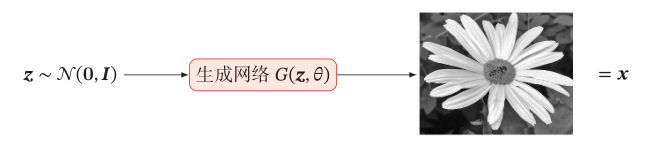# 生成对抗网络

• 判别网络：目标是尽量准确地判断一个样本是来自于真实数据还是由生成网络产生。
• 生成网络：目标是尽量生成判别网络无法区分来源的样本。

## 生成对抗网络的训练过程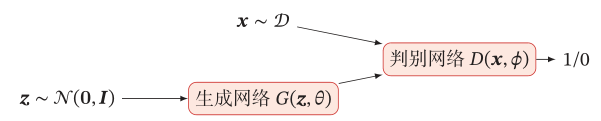### Step 1：固定生成网络，训练判别网络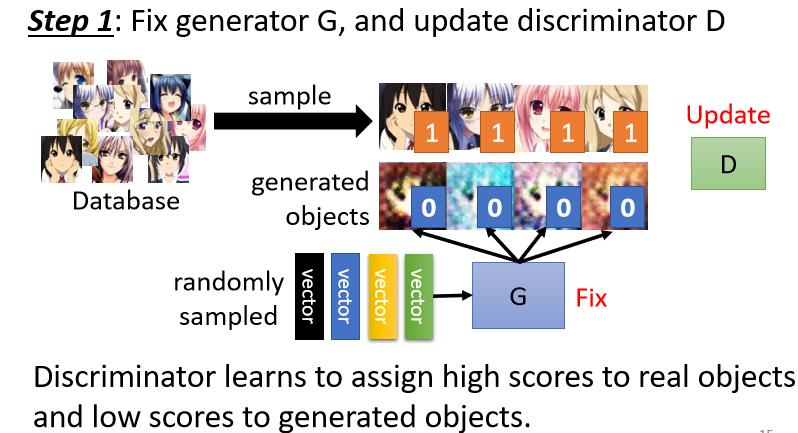• 分类问题：将真正的头像看作类别1，生成网络产生的图像看作类别2，然后利用这些图片训练一个分类器。
• 回归问题：训练判别网络看到真正的头像图片时输出1，看到生成网络生成的头像图片时输出0，来学习两者之间的差异。

### Step 2: 固定判别网络，训练生成网络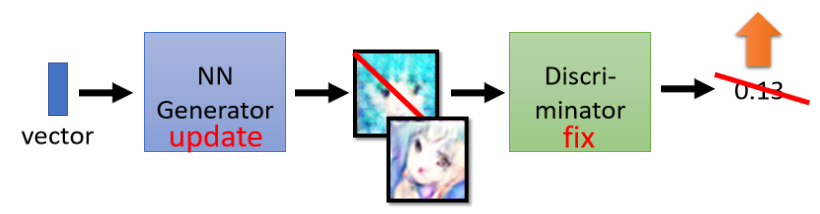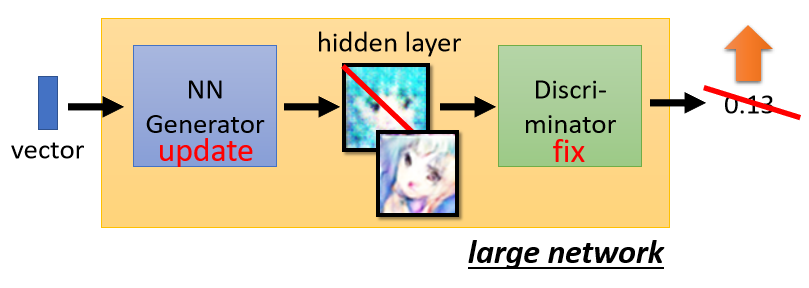## 生成网络

• 确定一个Loss Function
• 然后最小化Loss Function就可以了

[G^*=argmin_{G}Div(p_ heta, p_r) ag{1}
]

## 判别网络

[p(y=1|x)=D(x;phi) ag{2}
]

[p(y=0|x)=1 – D(x;phi) ag{3}
]

[min_{phi}- (mathbb{E}_x[ylog p(y=1|x)+(1-y)logp(y=0|x)]) ag{4}
]

[egin{aligned}
&max_phi V(D,G) \
=&max_{phi} mathbb{E}_{x sim p_r(x)}[logD(x;phi)]+mathbb{E}_{x’ sim p_ heta(x’)}[log(1-D(x’;phi))] \
=& max_{phi}mathbb{E_{x sim p_r(x)}}[logD(x;phi)] + mathbb{E}_{z sim p(z)}[log(1-D(G(z; heta); phi))]
end{aligned} ag{5}
]

• (mathbb{E}_{x sim p_r(x)})：是将真实的图像数据输入到判别网络中，得到一个分数再取(logD(x;phi))，为了让公式（5）中的目标函数越大，该项的值越大越好
• (mathbb{E}_{z sim p(z)})：是将生成的图像数据输入到判别网络中，得到一个分数再取(log(1-D(G(z; heta); phi)))，为了让公式（5）中的目标函数越大，该项的值越小越好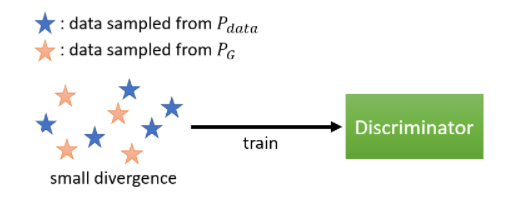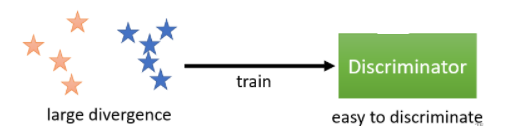[G^* = arg min_{G}max_{D}V(G,D) ag{6}
]

《神经网络与深度学习》 邱锡鹏